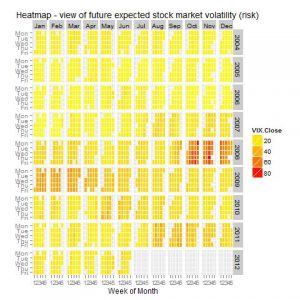# Using R as visualizing Volatility Index VIX

On September 22, 2003, the CBOE began disseminating price level information using revised methodology for the CBOE Volatility Index, VIX. A spreadsheet with more than 13 years of price history data using this new methodology is now available.

## VIX?

In 1993, the Chicago Board Options Exchange® (CBOE®) introduced the CBOE Volatility Index®, VIX®, and it quickly became the benchmark for stock market volatility. It is widely followed and has been cited in hundreds of news articles in the Wall Street Journal, Barron’s and other leading financial publications. Since volatility often signifies financial turmoil, VIX is often referred to as the “investor fear gauge”.
Source: http://www.cboe.com/micro/vix/faq.aspx#1

So, I found this example from there. I change it little bit. In this exampele it use whole time series you will found there. Now let’s look at closer this amazing approach…
``` #required library require(quantmod) require(ggplot2) require(reshape2) require(plyr) require(scales) `````` # download data source input <- read.table("http://www.cboe.com/publish/ScheduledTask/MktData/datahouse/vixcurrent.csv", header=TRUE, sep=",", na.strings="NA", dec=".", strip.white=TRUE) `````` # creating dataframe dat<-data.frame(date=index(VIX),VIX) #look what we get head(dat) `````` #date conversation input\$date <- as.Date(input\$Date, "%m/%d/%Y") `````` ## below some example do to that ## read in date/time info in format 'm/d/y h:m:s' ## dates <- c("02/27/92", "02/27/92", "01/14/92", "02/28/92", "02/01/92") ## times <- c("23:03:20", "22:29:56", "01:03:30", "18:21:03", "16:56:26") ## x <- paste(dates) ## y <- strptime(x, "%m/%d/%y") `````` # extract year input\$year<-as.numeric(as.POSIXlt(input\$date)\$year+1900) `````` # and  the month too OK input\$month<-as.numeric(as.POSIXlt(input\$date)\$mon+1) `````` # monts into right order and giving factor name input\$monthf<-factor(input\$month,levels=as.character(1:12),labels=c("Jan","Feb","Mar","Apr","May","Jun","Jul","Aug","Sep","Oct","Nov","Dec"),ordered=TRUE) `````` # week days input\$weekday = as.POSIXlt(input\$date)\$wday `````` # week day ordering and gibing factor name (weekdays) input\$weekdayf<-factor(input\$weekday,levels=rev(1:7),labels=rev(c("Mon","Tue","Wed","Thu","Fri","Sat","Sun")),ordered=TRUE) `````` # yearmonth input\$yearmonth<-as.yearmon(input\$date) input\$yearmonthf<-factor(input\$yearmonth) `````` # week of year by rows input\$week <- as.numeric(format(input\$date,"%W")) `````` # making week to start at number 1 input<-ddply(input,.(yearmonthf),transform,monthweek=1+week-min(week)) `````` # Ok, Now we are ready to plot `````` P<- ggplot(input, aes(monthweek, weekdayf, fill = VIX.Close)) + geom_tile(colour = "white") + facet_grid(year~monthf) + scale_fill_gradient(low="yellow", high="red") + opts(title = "Heatmap - view of future expected stock market volatility (risk)") + xlab("Week of Month") + ylab("") `````` #show the plot P `````` # save the picture # example - output graph to jpeg file jpeg("heatmaps.jpg") P dev.off() `````` ...and output will look like this:```That’s it,

Cheers, Marko